## 一维准晶功能梯度层合圆柱壳热电弹性精确解 1)

*中国农业大学理学院, 北京 100083

## EXACT THERMO-ELECTRO-ELASTIC SOLUTION OF FUNCTIONALLY GRADED MULTILAYERED ONE-DIMENSIONAL QUASICRYSTAL CYLINDRICAL SHELLS 1)

Li Yang*,, Qin Qinghua, Zhang Liangliang*, Gao Yang,*,2)

*College of Science, China Agricultural University, Beijing 100083, China

College of Engineering and Computer Science, Australian National University, Canberra 2601, Australia

 基金资助: 1)国家自然科学基金.  11972365国家自然科学基金.  51704015辽宁省教育厅科学研究经费.  L2019006

Received: 2020-04-18   Accepted: 2020-04-18   Online: 2020-09-18Abstract

Layered structures made of two and more materials with different properties can meet the needs of industrial development. However, the abrupt change of material properties at the interface of the laminated structures can easily cause some interface problems, such as stress concentration, interface cracks, and interface delamination phenomena. Functionally graded materials refer to utilize a continuously changing component gradient instead of the original sudden change interface, which can eliminate or weaken the abrupt change of the physical properties and then increase the bonding strength of the layered structures. In this paper, the research object is the functionally graded multilayered one-dimensional quasicrystal cylindrical shells. By virtue of the pseudo-Stroh formalism and the propagator matrix method, we establish the layered one-dimensional quasicrystal cylindrical shells model with the material parameters following the power-law type distribution along its radius direction, and obtain the exact thermo-electro-elastic solution of the functionally graded layered one-dimensional quasicrystal cylindrical shells with simply supported boundary condition. Numerical examples are carried out to investigate the influences of the exponential factor on temperature, electric, phason and phonon fields of the functionally graded layered one-dimensional quasicrystal cylindrical shells subjected to both inner and outer surfaces temperature variations, especially the effects on physical quantities at the inner and outer surfaces of the layered one-dimensional quasicrystal cylindrical shells. The obtained results indicate that: the exponential factor can change the distribution characteristic of material parameters, which can cause a significant influence on the physical quantities in the temperature, electric, phason, and phonon fields; by increasing the exponential factor, the deformation at the internal surface induced by temperature stimuli is reduced and the strength of the layered one-dimensional quasicrystal cylindrical shells is improved. The results obtained in this paper can provide a reliable theoretical basis for the design and manufacture of functionally graded layered one-dimensional quasicrystal cylindrical shells.

Keywords： functionally graded materials ; quasicrystals ; layered cylindrical shells ; thermo-electro-elastic coupling effect ; exact solution

Li Yang, Qin Qinghua, Zhang Liangliang, Gao Yang. EXACT THERMO-ELECTRO-ELASTIC SOLUTION OF FUNCTIONALLY GRADED MULTILAYERED ONE-DIMENSIONAL QUASICRYSTAL CYLINDRICAL SHELLS 1). Chinese Journal of Theoretical and Applied Mechanics[J], 2020, 52(5): 1286-1294 DOI:10.6052/0459-1879-20-126

## 1 基本方程

### 图1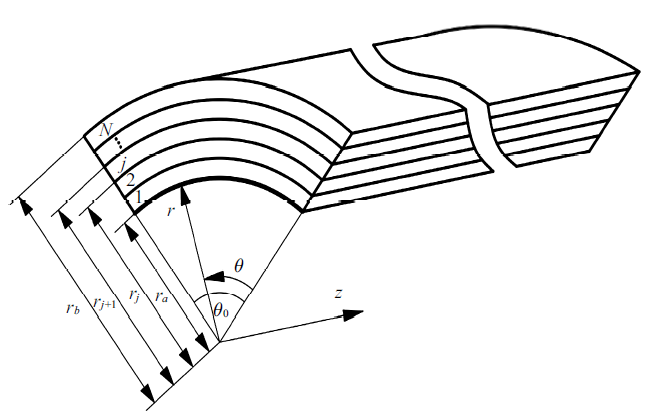Fig.1   Schematic of functionally graded layered 1Dquasicrystal cylindrical shells

$\begin{eqnarray} \label{eq1} \left. \begin{array}{ll} \sigma _{rr} =C_{33} \varepsilon _{rr} +C_{13} \varepsilon _{\theta \theta } +C_{23} \varepsilon _{zz} +R_3 w_{rr} -\\ \qquad e_{33} E_r -\beta _3 T \\ \sigma _{\theta \theta } =C_{13} \varepsilon _{rr} +C_{11} \varepsilon _{\theta \theta } +C_{12} \varepsilon _{zz} +R_1 w_{rr} -\\ \qquad e_{31} E_r -\beta _1 T \\ \sigma _{zz} =C_{23} \varepsilon _{rr} +C_{12} \varepsilon _{\theta \theta } +C_{22} \varepsilon _{zz} +R_2 w_{rr} -\\ \qquad e_{32} E_r -\beta _1 T \\ \sigma _{\theta z} =\sigma _{z\theta } =2C_{66} \varepsilon _{\theta z} \\ \sigma _{rz} =\sigma _{zr} =2C_{44} \varepsilon _{zr} +R_5 w_{rz} -e_{24} E_z \\ \sigma _{r\theta } =\sigma _{\theta r} =2C_{55} \varepsilon _{r\theta } +R_6 w_{r\theta } -e_{15} E_\theta \\ H_{rr} =R_3 \varepsilon _{rr} +R_1 \varepsilon _{\theta \theta } +R_2 \varepsilon _{zz} +K_3 w_{rr} -d_{33} E_r \\ H_{rz} =2R_5 \varepsilon _{zr} +K_2 w_{rz} -d_{24} E_z \\ H_{r\theta } =2R_6 \varepsilon _{r\theta } +K_1 w_{r\theta } -d_{15} E_\theta \\ D_r =e_{33} \varepsilon _{rr} +e_{31} \varepsilon _{\theta \theta } +e_{32} \varepsilon _{zz} +d_{33} w_{rr} +\\ \qquad \xi _{33} E_r +p_3 T \\ D_\theta =2e_{15} \varepsilon _{r\theta } +d_{15} w_{r\theta } +\xi _{11} E_\theta \\ D_z =2e_{24} \varepsilon _{zr} +d_{24} w_{rz} +\xi _{11} E_z \\ \end{array} \right\} \end{eqnarray}$

$\begin{eqnarray} \label{eq2} \left. \begin{array}{ll} \sigma _{rr,r} +{\sigma _{r\theta ,\theta } } / r+\sigma _{rz,z} +{\left( {\sigma _{rr} -\sigma _{\theta \theta } } \right)} / r=0 \\ \sigma _{r\theta ,r} +{\sigma _{\theta \theta ,\theta } } / r+\sigma _{\theta z,z} +{2\sigma _{r\theta } } / r=0 \\ \sigma _{rz,r} +{\sigma _{\theta z,\theta } } / r+\sigma _{zz,z} +{\sigma _{rz} } / r=0 \\ H_{rr,r} +{H_{r\theta ,\theta } } / r+H_{rz,z} +{H_{rr} } / r=0 \\ D_{r,r} +{D_{\theta ,\theta } } / r+D_{z,z} +{D_r } / r=0 \\ q_{r,r} +{q_{\theta ,\theta } } / r+q_{z,z} +{q_r } / r=0 \\ \end{array} \right\} \end{eqnarray}$

$\begin{eqnarray} \label{eq3} q_r =-k_{11} T_{,r},\ \ q_\theta =-k_{22} T_{,\theta} / r,\ \ q_z =-k_{33} T_{,z} \end{eqnarray}$

$\begin{eqnarray} \label{eq4} \left. \begin{array}{ll} \varepsilon _{rr} =u_{r,r} , \ \ \varepsilon _{\theta \theta } ={\left( {u_{\theta,\theta } +u_r } \right)} / r, \ \ \varepsilon _{zz} =u_{z,z}\\ \varepsilon _{\theta z} =\varepsilon _{z\theta } =0.5\left( {u_{\theta ,z} +{u_{z,\theta } } / r} \right) \\ \varepsilon _{rz} =\varepsilon _{zr} =0.5\left( {u_{z,r} +u_{r,z} } \right)\\ \varepsilon _{\theta r} =\varepsilon _{r\theta } =0.5\left( {{u_{r,\theta }} / r+u_{\theta ,r} -{u_\theta } / r} \right) \\ w_{rr} =w_{r,r} , \ \ w_{r\theta } =w_{r,\theta}/r, \ \ w_{rz} =w_{r,z} \\ E_r =-\phi _{,r}, \ \ E_\theta =-\phi _{,\theta } / r, \ \ E_z =-\phi _{,z} \\ \end{array} \right\} \end{eqnarray}$

$\begin{eqnarray} \label{eq5} \theta =0,\ \ \theta _0;\ \ \ u_r =w_r =\phi =T=0 \end{eqnarray}$

$\begin{eqnarray} \label{eq6} \left. \begin{array}{ll} C_{kl} (r)=C_{kl}^0 (r/r_j )^\alpha , \ \ R_{kl} (r)=R_{kl}^0 (r/r_j )^\alpha \\ K_{kl} (r)=K_{kl}^0 (r/r_j )^\alpha , \ \ e_{kl} (r)=e_{kl}^0 (r/r_j )^\alpha \\ d_{kl} (r)=d_{kl}^0 (r/r_j )^\alpha , \ \ \xi _{ll} (r)=\xi _{ll}^0 (r/r_j )^\alpha \ \\ \beta _k (r)=\beta _k^0 (r/r_j )^\alpha , \ \ k_{ll} (r)=k_{ll}^0 (r/r_j )^\alpha \\ p_3 (r)=p_3^0 (r/r_j )^\alpha \\ \end{array} \right\} \end{eqnarray}$

## 2 单层圆柱壳的热电弹性精确解

### 2.1 温度场的精确解

$\begin{eqnarray} \label{eq7} T=\bar{{T}}\left( r \right)\sin \left( {p\theta } \right)=f\rho ^\eta \sin \left( {p\theta } \right) \end{eqnarray}$

$\begin{eqnarray} \label{eq8} \left( {k_{11}^0 \eta ^2+k_{11}^0 \alpha \eta -k_{22}^0 p^2} \right)f=0 \end{eqnarray}$

$\begin{eqnarray} \label{eq9} \eta _1 =\frac{-\alpha +\sqrt {\alpha ^2+4k_{22}^0 p^2/k_{11}^0 }}{2}, \ \ \eta _2 =-\eta _1 -\alpha \end{eqnarray}$

$\begin{eqnarray} \label{eq10} \left[ {{\begin{array}{cc} 0 & {{-1} / {k_{11}^0 }} \\ {-p^2k_{22}^0 } & {-\alpha } \\ \end{array} }} \right]\left[ {{\begin{array}{c} f \\ g \\ \end{array} }} \right]=\eta \left[ {{\begin{array}{c} f \\ g \\ \end{array} }} \right] \end{eqnarray}$

$\begin{eqnarray} \label{eq11} \left[ {{\begin{array}{*{20}c} {\bar{{T}}} \\ {r\bar{{q}}_r } \\ \end{array} }} \right]=\left[ {{\begin{array}{*{20}c} 1 & 0 \\ 0 & {\rho ^\alpha } \\ \end{array} }} \right]\left[ {{\begin{array}{*{20}c} {f_1 } & {f_2 } \\ {g_1 } & {g_2 } \\ \end{array} }} \right]\left[ {{\begin{array}{*{20}c} {\rho ^{\eta _1 }} & 0 \\ 0 & {\rho ^{\eta _2 }} \\ \end{array} }} \right]\left[ {{\begin{array}{*{20}c} {\chi _1 } \\ {\chi _2 } \\ \end{array} }} \right] \end{eqnarray}$

### 2.2 电弹耦合场的精确解

$\begin{eqnarray} \label{eq12} && { u}=\left[ {{\begin{array}{*{20}c} {u_r } \\ {u_\theta } \\ {u_z } \\ {w_r } \\ \phi \\ \end{array} }} \right]=\left[ {{\begin{array}{*{20}c} {\bar{{u}}_r \sin \left( {p\theta } \right)} \\ {\bar{{u}}_\theta \cos \left( {p\theta } \right)} \\ {\bar{{u}}_z \cos \left( {p\theta } \right)} \\ {\bar{{w}}_r \sin \left( {p\theta } \right)} \\ {\bar{{\phi }}\sin \left( {p\theta } \right)} \\ \end{array} }} \right]= \rho ^s\left[ {{\begin{array}{*{20}c} {a_1 \sin \left( {p\theta } \right)} \\ {a_2 \cos \left( {p\theta } \right)} \\ {a_3 \cos \left( {p\theta } \right)} \\ {a_4 \sin \left( {p\theta } \right)} \\ {a_5 \sin \left( {p\theta } \right)} \\ \end{array} }} \right]+ \\ &&\qquad f\rho ^{1+\eta }\left[ {{\begin{array}{*{20}c} {c_1 \sin \left( {p\theta } \right)} \\ {c_2 \cos \left( {p\theta } \right)} \\ {c_3 \cos \left( {p\theta } \right)} \\ {c_4 \sin \left( {p\theta } \right)} \\ {c_5 \sin \left( {p\theta } \right)} \\ \end{array} }} \right] \end{eqnarray}$

$\begin{eqnarray} \label{eq13} && r{ t}=r\left[ {{\begin{array}{*{20}c} {\sigma _{rr} } \\ {\sigma _{\theta r} } \\ {\sigma _{zr} } \\ {H_{rr} } \\ {D_r } \\ \end{array} }} \right]=r\left[ {{\begin{array}{*{20}c} {\bar{{\sigma }}_{rr} \sin \left( {p\theta } \right)} \\ {\bar{{\sigma }}_{\theta r} \cos \left( {p\theta } \right)} \\ {\bar{{\sigma }}_{zr} \cos \left( {p\theta } \right)} \\ {\bar{{H}}_{rr} \sin \left( {p\theta } \right)} \\ {\bar{{D}}_r \sin \left( {p\theta } \right)} \\ \end{array} }} \right] =\rho ^{s+\alpha }\left[ {{\begin{array}{*{20}c} {b_1 \sin \left( {p\theta } \right)} \\ {b_2 \cos \left( {p\theta } \right)} \\ {b_3 \cos \left( {p\theta } \right)} \\ {b_4 \sin \left( {p\theta } \right)} \\ {b_5 \sin \left( {p\theta } \right)} \\ \end{array} }} \right]+ \\ &&\qquad f\rho ^{1+\eta +\alpha }\left[ {{\begin{array}{*{20}c} {d_1 \sin \left( {p\theta } \right)} \\ {d_2 \cos \left( {p\theta } \right)} \\ {d_3 \cos \left( {p\theta } \right)} \\ {d_4 \sin \left( {p\theta } \right)} \\ {d_5 \sin \left( {p\theta } \right)} \\ \end{array} }} \right] \end{eqnarray}$

$\begin{eqnarray} \label{eq14} \left. \begin{array}{ll} { a}=\left[ {a_1 ,a_2 ,a_3 ,a_4 ,a_5 } \right]^{\rm T}, { b}=\left[ {b_1 ,b_2 ,b_3 ,b_4 ,b_5 } \right]^{\rm T} \\ { c}=\left[ {c_1 ,c_2 ,c_3 ,c_4 ,c_5 } \right]^{\rm T}, { d}=\left[ {d_1 ,d_2 ,d_3 ,d_4 ,d_5 } \right]^{\rm T} \\ \end{array} \right\} \end{eqnarray}$

$\begin{eqnarray} \label{eq15} \left. \begin{array}{ll} { b}=\left( {-{ P}^{\rm T}+s{ T}} \right){ a} \\ { d} \ \ =\left[ {-{ P}^{\rm T}+\left( {1+\eta } \right){ T}} \right]{ c}-{ \beta }_2 \\ \end{array} \right\} \end{eqnarray}$

$\begin{eqnarray} \label{eq16} \left. \begin{array}{ll} \left[ {{ Q}-\alpha { P}^T+s\left( {{ P}-{ P}^T+\alpha { T}} \right)+s^2{ T}} \right]{ a}={\bf 0} \\ \left[{ Q}-\alpha { P}^T+\left( {1+\eta } \right)\left( {{ P}-{ P}^T+\alpha { T}} \right)+ \\ \qquad \left( {1+\eta } \right)^2{ T} \right]{ c}={ \beta }_1 +\left( {1+\eta +\alpha } \right){ \beta }_2 \\ \end{array} \right\} \end{eqnarray}$

$\begin{eqnarray} \label{eq17} \left. \begin{array}{ll} { Q}=\left[ {{\begin{array}{c@{\ \ \ }c@{\ \ \ }c@{\ \ \ }c@{\ \ \ }c} {-\left( {C_{55}^0 p^2+C_{11}^0 } \right)} & {\left( {C_{55}^0 +C_{11}^0 } \right)p} & 0 & {-R_6^0 p^2} & {-e_{15}^0 p^2} \\ {\left( {C_{55}^0 +C_{11}^0 } \right)p} & {-\left( {C_{11}^0 p^2+C_{55}^0 } \right)} & 0 & {R_6^0 p} & {e_{15}^0 p} \\ 0 & 0 & {-C_{66}^0 p^2} & 0 & 0 \\ {-R_6^0 p^2} & {R_6^0 p} & 0 & {-K_1^0 p^2} & {-d_{15}^0 p^2} \\ {-e_{15}^0 p^2} & {e_{15}^0 p} & 0 & {-d_{15}^0 p^2} & {\xi _{11}^0 p^2} \\ \end{array} }} \right], \ \ { P}=\left[ {{\begin{array}{c@{\ \ \ }c@{\ \ \ }c@{\ \ \ }c@{\ \ \ }c} {-C_{13}^0 } & {-C_{55}^0 p} & 0 & {-R_1^0 } & {-e_{31}^0 } \\ {C_{13}^0 p} & {C_{55}^0 } & 0 & {R_1^0 p} & {e_{31}^0 p} \\ 0 & 0 & 0 & 0 & 0 \\ 0 & {-R_6^0 p} & 0 & 0 & 0 \\ 0 & {-e_{15}^0 p} & 0 & 0 & 0 \\ \end{array} }} \right]\\[10mm] { T}=\left[ {{\begin{array}{{c@{\ \ \ }c@{\ \ \ }c@{\ \ \ }c@{\ \ \ }c}} {C_{33}^0 } & 0 & 0 & {R_3^0 } & {e_{33}^0 } \\ 0 & {C_{55}^0 } & 0 & 0 & 0 \\ 0 & 0 & {C_{44}^0 } & 0 & 0 \\ {R_3^0 } & 0 & 0 & {K_3^0 } & {d_{33}^0 } \\ {e_{33}^0 } & 0 & 0 & {d_{33}^0 } & {-\xi _{33}^0 } \\ \end{array} }} \right], \ \ { \beta }_1 =r_j \left[ {{\begin{array}{*{20}c} {-\beta _1^0 } \\ {\beta _1^0 p} \\ 0 \\ 0 \\ 0 \\ \end{array} }} \right], \ \ { \beta }_2 =r_j \left[ {{\begin{array}{*{20}c} {\beta _3^0 } \\ 0 \\ 0 \\ 0 \\ {-p_3^0 } \\ \end{array} }} \right] \\ \end{array} \right\} \end{eqnarray}$

$\begin{eqnarray} \label{eq18} { N}\left[ {\begin{array}{l} { a} \\ { b} \\ \end{array}} \right]=s\left[ {\begin{array}{l} { a} \\ { b} \\ \end{array}} \right], \ \ { N}\left[ {\begin{array}{l} { c} \\ { d} \\ \end{array}} \right]=\left( {1+\eta } \right)\left[ {\begin{array}{l} { c} \\ { d} \\ \end{array}} \right]+{ \beta } \end{eqnarray}$

$\begin{eqnarray} \label{eq19} \begin{array}{l} { N}=\left[ {{\begin{array}{*{20}c} {{ T}^{-1}{ P}^{\rm T}} & {{ T}^{-1}} \\ {-{ Q}-{ PT}^{-1}{ P}^{\rm T}} & {-{ PT}^{-1}-\alpha { I}} \\ \end{array} }} \right] \\ { \beta }=-\left[ {{\begin{array}{*{20}c} {\bf 0} & {{ T}^{-1}} \\ { I} & {-{ PT}^{-1}} \\ \end{array} }} \right]\left[ {{\begin{array}{*{20}c} {{ \beta }_1 } \\ {{ \beta }_2 } \\ \end{array} }} \right] \\ \end{array} \end{eqnarray}$

$\begin{eqnarray} \label{eq20} && \left[ {{\begin{array}{*{20}c} {{\bar{{ u}}}} \\ {r{\bar{{ t}}}} \\ \end{array} }} \right]=\left[ {{\begin{array}{*{20}c} { I} & {\bf 0} \\ {\bf 0} & {\rho ^\alpha { I}} \\ \end{array} }} \right]\left[ {{\begin{array}{*{20}c} {{ A}_1 } & {{ A}_2 } \\ {{ B}_1 } & {{ B}_2 } \\ \end{array} }} \right]\left\langle {\rho ^{s\ast }} \right\rangle \left[ {\begin{array}{l} { K}_1 \\ { K}_2 \\ \end{array}} \right]+ \left[ {{\begin{array}{*{20}c} {\rho { I}} & {\bf 0} \\ {\bf 0} & {\rho ^{1+\alpha }{ I}} \\ \end{array} }} \right]\cdot \\&&\qquad \left[ {{\begin{array}{*{20}c} {{ c}_1 } & {{ c}_2 } \\ {{ d}_1 } & {{ d}_2 } \\ \end{array} }} \right]\left[ {{\begin{array}{*{20}c} {\rho ^{\eta _1 }} & 0 \\ 0 & {\rho ^{\eta _2 }} \\ \end{array} }} \right]\left[ {{\begin{array}{*{20}c} {f_1 } & 0 \\ 0 & {f_2 } \\ \end{array} }} \right]\left[ {{\begin{array}{*{20}c} {\chi _1 } \\ {\chi _2 } \\ \end{array} }} \right] \end{eqnarray}$

$\begin{eqnarray} \label{eq21} \left. \begin{array}{ll} \left[ \begin{array}{*{20}c} {{\bar{{ u}}}} & {r{\bar{{ t}}}} \\ \end{array} \right]^{\rm T}=\left[ \bar{{u}}_r ,\ \ \bar{{u}}_\theta ,\ \ \bar{{u}}_z ,\ \ \bar{{w}}_r , \ \ \bar{{\phi }}, \ \ r\bar{{\sigma }}_{rr} , \ \ r\bar{{\sigma }}_{\theta r} , \\\qquad r\bar{{\sigma }}_{zr} ,\ \ r\bar{{H}}_{rr} , \ \ r\bar{{D}}_r \right]^{\rm T} \\ { A}_1 =\left[ {{ a}_1 , \ \ { a}_2 , \ \ { a}_3 , \ \ { a}_4 , \ \ { a}_5 } \right]\\ { A}_2 =\left[ { \ \ \ { a}_6 , \ \ { a}_7 , \ \ { a}_8 , \ \ { a}_9 , \ \ { a}_{10} } \right] \\ { B}_1 =\left[ {{ b}_1 , \ \ { b}_2 , \ \ { b}_3 , \ \ { b}_4 , \ \ { b}_5 } \right]\\{ B}_2 =\left[ {{ b}_6 , \ \ { b}_7 , \ \ { b}_8 , \ \ { b}_9 , \ \ { b}_{10} } \right] \\ \left\langle {\rho ^{s^\ast }} \right\rangle = \mbox{diag}\left[ \rho ^{s_1 }, \ \ \rho ^{s_2 }, \ \ \rho ^{s_3 }, \ \ \rho ^{s_4 },\ \ \rho ^{s_5 }, \ \ \rho ^{-s_1 -\alpha }, \\\qquad \rho ^{-s_2 -\alpha }, \ \ \rho ^{-s_3 -\alpha }, \ \ \rho ^{-s_4 -\alpha },\ \ \rho ^{-s_5 -\alpha } \right] \\ \end{array} \right\} \end{eqnarray}$

## 3 层合圆柱壳的热电弹性精确解

$\begin{eqnarray} \label{eq22} && \left[ {{\begin{array}{*{20}c} {\chi _1 } \\ {\chi _2 } \\ \end{array} }} \right]_j =\left[ {{\begin{array}{*{20}c} {f_1 } & {f_2 } \\ {g_1 } & {g_2 } \\ \end{array} }} \right]^{-1}\left[ {{\begin{array}{*{20}c} {\bar{{T}}} \\ {r_j \bar{{q}}_r } \\ \end{array} }} \right]_{r_j } =\left[ {{\begin{array}{*{20}c} {(r_{j+1} /r_j )^{-\eta _1 }} & 0 \\ 0 & {(r_{j+1} /r_j )^{-\eta _2 }} \\ \end{array} }} \right]\cdot \\&&\qquad \left[ {{\begin{array}{*{20}c} {f_1 } & {f_2 } \\ {g_1 } & {g_2 } \\ \end{array} }} \right]^{-1}\left[ {{\begin{array}{*{20}c} 1 & 0 \\ 0 & {(r_{j+1} /r_j )^{-\alpha }} \\ \end{array} }} \right]\left[ {{\begin{array}{*{20}c} {\bar{{T}}} \\ {r_{j+1} \bar{{q}}_r } \\ \end{array} }} \right]_{r_{j+1} } \end{eqnarray}$

$\begin{eqnarray} \label{eq23} \left[ {{\begin{array}{*{20}c} {\bar{{T}}} \\ {r\bar{{q}}_r } \\ \end{array} }} \right]={ T}_j (r/r_j )\left[ {{\begin{array}{*{20}c} {\bar{{T}}} \\ {r_j \bar{{q}}_r } \\ \end{array} }} \right]_{r_j } \end{eqnarray}$

$\begin{eqnarray} \label{eq24} && { T}_j (r/r_j )=\left[ {{\begin{array}{*{20}c} 1 & 0 \\ 0 & {(r/r_j )^\alpha } \\ \end{array} }} \right]\left[ {{\begin{array}{*{20}c} {f_1 } & {f_2 } \\ {g_1 } & {g_2 } \\ \end{array} }} \right]\cdot \\&&\qquad \left[ {{\begin{array}{*{20}c} {(r/r_j )^{\eta _1 }} & 0 \\ 0 & {(r/r_j )^{\eta _2 }} \\ \end{array} }} \right]\left[ {{\begin{array}{*{20}c} {f_1 } & {f_2 } \\ {g_1 } & {g_2 } \\ \end{array} }} \right]^{-1} \end{eqnarray}$

$\begin{eqnarray} \label{eq25} && \left[ {\begin{array}{l} { k}_1 \\ { k}_2 \\ \end{array}} \right]_j =\left[ {{\begin{array}{*{20}c} {{ a}_1 } & {{ a}_2 } \\ {{ b}_1 } & {{ b}_2 } \\ \end{array} }} \right]^{-1}\left[ {{\begin{array}{*{20}c} {{\bar{{ u}}}} \\ {r_j {\bar{{ t}}}} \\ \end{array} }} \right]_{r_j } -\left[ {{\begin{array}{*{20}c} {{ a}_1 } & {{ a}_2 } \\ {{ b}_1 } & {{ b}_2 } \\ \end{array} }} \right]^{-1}\left[ {{\begin{array}{*{20}c} {{ c}_1 } & {{ c}_2 } \\ {{ d}_1 } & {{ d}_2 } \\ \end{array} }} \right]\cdot \\&&\qquad \left[ {{\begin{array}{*{20}c} {f_1 } & 0 \\ 0 & {f_2 } \\ \end{array} }} \right]\left[ {{\begin{array}{*{20}c} {\chi _1 } \\ {\chi _2 } \\ \end{array} }} \right]_j =\left\langle {(r_{j+1} /r_j )^{-s\ast }} \right\rangle \left[ {{\begin{array}{*{20}c} {{ a}_1 } & {{ a}_2 } \\ {{ b}_1 } & {{ b}_2 } \\ \end{array} }} \right]^{-1}\cdot \\&&\qquad \left[ {{\begin{array}{*{20}c} { i} & {\bf 0} \\ {\bf 0} & {(r_{j+1} /r_j )^{-\alpha }{ i}} \\ \end{array} }} \right]\left[ {{\begin{array}{*{20}c} {{\bar{{ u}}}} \\ {r_{j+1} {\bar{{ t}}}} \\ \end{array} }} \right]_{r_{j+1} } -\left\langle {(r_{j+1} /r_j )^{-s\ast }} \right\rangle\cdot \\&&\qquad\left[ {{\begin{array}{*{20}c} {{ a}_1 } & {{ a}_2 } \\ {{ b}_1 } & {{ b}_2 } \\ \end{array} }} \right]^{-1} \left[ {{\begin{array}{*{20}c} { i} & {\bf 0} \\ {\bf 0} & {(r_{j+1} /r_j )^{-\alpha }{ i}} \\ \end{array} }} \right]\cdot \\&&\qquad\left[ {{\begin{array}{*{20}c} {(r_{j+1} /r_j ){ i}} & {\bf 0} \\ {\bf 0} & {(r_{j+1} /r_j )^{1+\alpha }{ i}} \\ \end{array} }} \right]\left[ {{\begin{array}{*{20}c} {{ c}_1 } & {{ c}_2 } \\ {{ d}_1 } & {{ d}_2 } \\ \end{array} }} \right]\cdot \\&&\qquad \left[ {{\begin{array}{*{20}c} {(r_{j+1} /r_j )^{\eta _1 }} & 0 \\ 0 & {(r_{j+1} /r_j )^{\eta _2 }} \\ \end{array} }} \right]\left[ {{\begin{array}{*{20}c} {f_1 } & 0 \\ 0 & {f_2 } \\ \end{array} }} \right]\left[ {{\begin{array}{*{20}c} {\chi _1 } \\ {\chi _2 } \\ \end{array} }} \right]_j \end{eqnarray}$

$\begin{eqnarray} \label{eq26} \left[ {{\begin{array}{*{20}c} {{\bar{{ u}}}} \\ {r{\bar{{ t}}}} \\ \end{array} }} \right]={ E}_j (r/r_j )\left[ {{\begin{array}{*{20}c} {{\bar{{ u}}}} \\ {r_j {\bar{{ t}}}} \\ \end{array} }} \right]_{r_j } +{ S}_j (r/r_j )\left[ {{\begin{array}{*{20}c} {\bar{{T}}} \\ {r_j \bar{{q}}_r } \\ \end{array} }} \right]_{r_j } \end{eqnarray}$

$\begin{eqnarray} \label{eq27} \left. \begin{array}{ll} { E}_j (r/r_j )=\left[ {{\begin{array}{*{20}c} { I} & {\bf 0} \\ {\bf 0} & {(r/r_j )^\alpha { I}} \\ \end{array} }} \right]\left[ {{\begin{array}{*{20}c} {{ A}_1 } & {{ A}_2 } \\ {{ B}_1 } & {{ B}_2 } \\ \end{array} }} \right]\left\langle {(r/r_j )^{s\ast }} \right\rangle\cdot\\\qquad \left[ {{\begin{array}{*{20}c} {{ A}_1 } & {{ A}_2 } \\ {{ B}_1 } & {{ B}_2 } \\ \end{array} }} \right]^{-1} \\ { S}_j (r/r_j )=\left[ {{\begin{array}{*{20}c} {(r/r_j ){ I}} & {\bf 0} \\ {\bf 0} & {(r/r_j )^{1+\alpha }{ I}} \\ \end{array} }} \right]\left[ {{\begin{array}{*{20}c} {{ c}_1 } & {{ c}_2 } \\ {{ d}_1 } & {{ d}_2 } \\ \end{array} }} \right]\\ \qquad\left[ {{\begin{array}{*{20}c} {(r/r_j )^{\eta _1 }} & 0 \\ 0 & {(r/r_j )^{\eta _2 }} \\ \end{array} }} \right] \left[ {{\begin{array}{*{20}c} {f_1 } & 0 \\ 0 & {f_2 } \\ \end{array} }} \right]\left[ {{\begin{array}{*{20}c} {f_1 } & {f_2 } \\ {g_1 } & {g_2 } \\ \end{array} }} \right]^{-1}-\\ \qquad{ E}_j (r/r_j )\left[ {{\begin{array}{*{20}c} {{ c}_1 } & {{ c}_2 } \\ {{ d}_1 } & {{ d}_2 } \\ \end{array} }} \right]\left[ {{\begin{array}{*{20}c} {f_1 } & 0 \\ 0 & {f_2 } \\ \end{array} }} \right]\left[ {{\begin{array}{*{20}c} {f_1 } & {f_2 } \\ {g_1 } & {g_2 } \\ \end{array} }} \right]^{-1} \\ \end{array} \right\} \end{eqnarray}$

$\begin{eqnarray} \label{eq28} &&\left[ \begin{array}{*{20}c} {{\bar{{ u}}}} \\ {r_b {\bar{{ t}}}} \\ {\bar{{T}}} \\ {r_b \bar{{q}}_r } \\ \end{array} \right]_{r_b } ={ G}_N (\delta _N ){ G}_{N-1} (\delta _{N-1} )\cdot \cdot \cdot { G}_2 (\delta _2 ){ G}_1 (\delta _1 )\cdot \\&&\qquad\left[ {{\begin{array}{*{20}c} {{\bar{{ u}}}} \\ {r_a {\bar{{ t}}}} \\ {\bar{{T}}} \\ {r_a \bar{{q}}_r } \\ \end{array} }} \right]_{r_a } \end{eqnarray}$

${ G}_j (\delta _j )=\left[ {{\begin{array}{c@{\quad }c} {{ E}_j (\delta _j )} & {{ S}_j (\delta _j )} \\ {\bf 0} & {{ T}_j (\delta _j )} \\ \end{array} }} \right]$

## 4 数值算例

Table 1  Material Constants tabular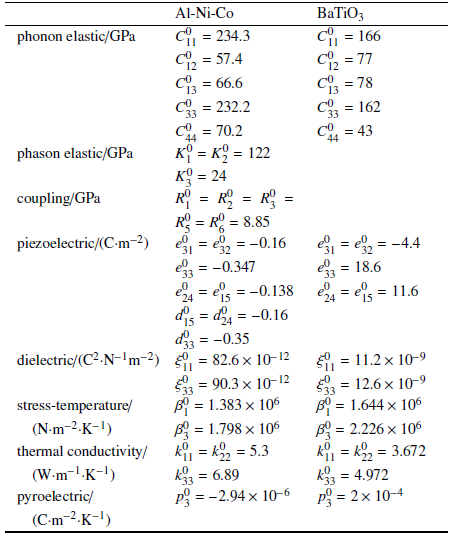### 图2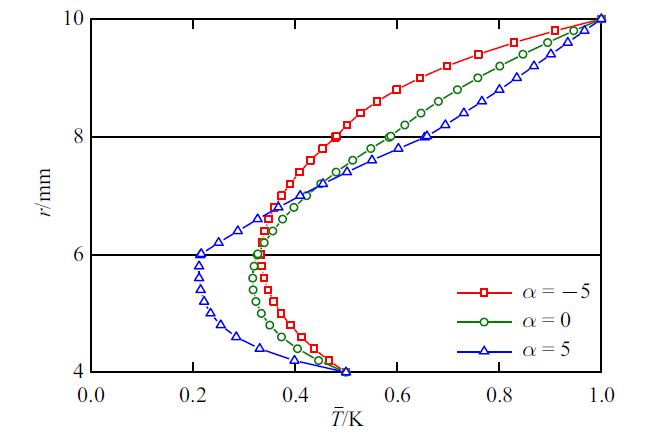Fig.2   Influence of exponential factor on temperature

### 图3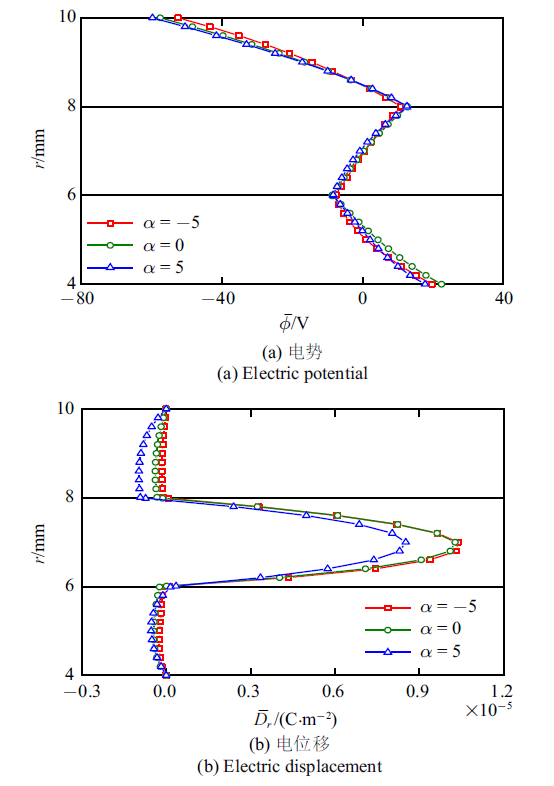Fig.3   Influence of exponential factor on electric field

### 图4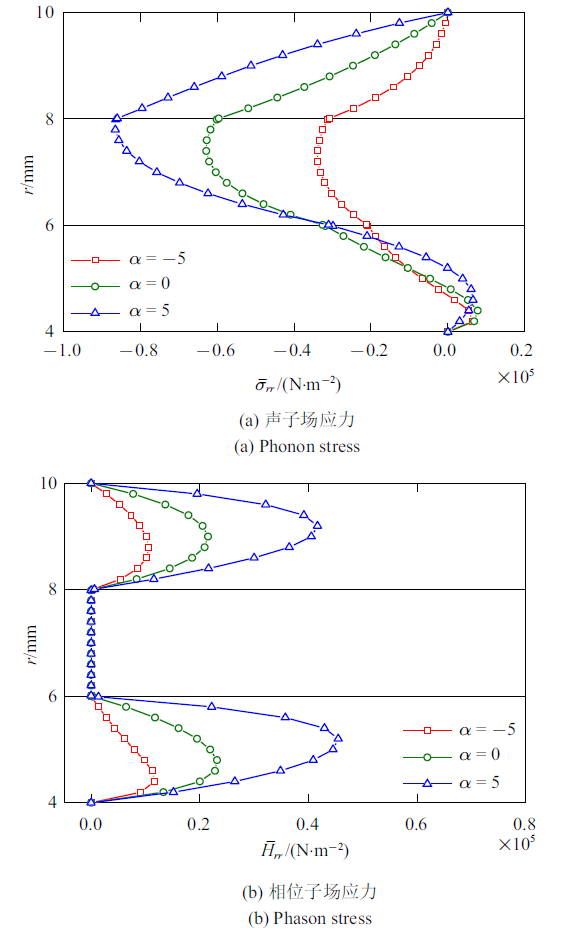Fig.4   Influence of exponential factor on phonon and phason stresses

### 图5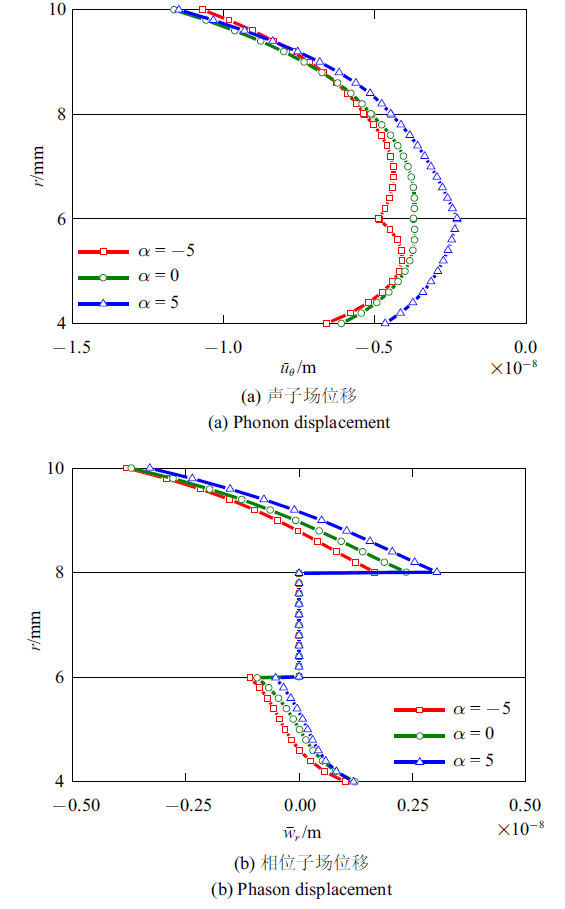Fig.5   Influence of exponential factor on phonon and phason displacements

## 5 结论

(1) 指数因子的变化影响层合圆柱壳的材料性质分布情况, 进而对温度、电势、电位移、声子场和相位子场应力和位移产生较大的影响.

(2) 随着指数因子的增大, 径向声子场应力增大, 且最大值出现在层合圆柱壳外层. 外层中较大的应力易于被外层凸面分散, 从而提升层合圆柱壳的承载能力.

(3) 随着指数因子的增大, 层合圆柱壳外表面周向声子场位移略有增大, 内表面周向声子场位移减小. 此外, 周向位移在界面处更为光滑, 可有效减缓层合圆柱壳在界面处出现开裂和分层等现象.

## 参考文献 原文顺序 文献年度倒序 文中引用次数倒序 被引期刊影响因子

( Ye Tiangui, Jin Guoyong, Liu Zhigang.

A spectral-differential quadrature method for 3-d vibration analysis of multilayered shells

Chinese Journal of Theoretical and Applied Mechanics, 2018,50(4):847-852 (in Chinese))

( Zhong Zheng, Wu Linzhi, Chen Weiqiu.

Progress in the study of mechanics problems of functionally graded materials and structures

Advances in Mechanics, 2010,40(5):528-541 (in Chinese))

( Ke Liaoliang, Wang Yuesheng.

Progress in some basic problems on contact mechanics of functionally graded materials

Chin Sci Bull, 2015,60:1565-1573 (in Chinese))

( Zheng Baojing, Liang Yu, Gao Xiaowei, et al.

Analysis for dynamic response of functionally graded materials using pod based reduced order model

Chinese Journal of Theoretical and Applied Mechanics, 2018,50(4):787-797 (in Chinese))

( Yang Jianpeng, Wang Huiming.

Chemomechanical analysis of a functionally graded spherical hydrogel

Chinese Journal of Theoretical and Applied Mechanics, 2019,51(4):1054-1063 (in Chinese))

Yang QQ, Gao CF.

Reduction of the stress concentration around an elliptic hole by using a functionally graded layer

Acta Mechanica, 2016,227(9):2427-2437

Yang B, Chen WQ, Ding HJ.

Approximate elasticity solutions for functionally graded circular plates subject to a concentrated force at the center

Mathematics and Mechanics of Solids, 2014,19(3):277-288

Pan E, Han F.

Exact solution for functionally graded and layered magneto-electro-elastic plates

International Journal of Engineering Science, 2005,43(3-4):321-339

Shechtman D, Blech I, Gratias D, et al.

Metallic phase with long-range orientational order and no translational symmetry

Physical Review Letters, 1984,53(20):1951-1953

( Fan Tianyou.

Mathematical theory of elasticity and defects of quasicrystals

Advances in Mechanics, 2000,30(2):161-174 (in Chinese))

Bak P.

Symmetry, stability, and elastic properties of icosahedral incommensurate crystals

Physical Review B, 1985,32(9):5764-5772

Gao Y, Ricoeur A.

Three-dimensional Green's functions for two-dimensional quasi-crystal bimaterials

Proceedings of the Royal Society A, 2011,467(2133):2622-2642

Jaric M.

Introduction to Quasicrystals

Elsevier, 2012

Fan TY.

A study on the specific heat of a one-dimensional hexagonal quasicrystal

Journal of Physics-Condensed Matter, 1999,11(45):513-517

Dubois JM.

Properties- and applications of quasicrystals and complex metallic alloys

Chemical Society Reviews, 2012,41(20):6760-6777

URL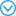This article aims at an account of what is known about the potential for applications of quasicrystals and related compounds, the so-called family of Complex Metallic Alloys (CMAsdouble dagger). Attention is focused at aluminium-based CMAs, which comprise a large number of crystalline compounds and quasicrystals made of aluminium alloyed with transition metals (like Fe or Cu) or normal metals like Mg. Depending on composition, the structural complexity varies from a few atoms per unit cell up to thousands of atoms. Quasicrystals appear then as CMAs of ultimate complexity and exhibit a lattice that shows no periodicity anymore in the usual 3-dimensional space. Properties change dramatically with lattice complexity and turn the metal-type behaviour of simple Al-based crystals into a far more complex behaviour, with a fingerprint of semi-conductors that may be exploited in various applications, potential or realised. An account of the ones known to the author is given in the light of the relevant properties, namely light absorption, reduced adhesion and friction, heat insulation, reinforcement of composites for mechanical devices, and few more exotic ones. The role played by the search for applications of quasicrystals in the development of the field is briefly addressed in the concluding section.

Maugin GA.

A note on the thermo-mechanics of elastic quasi-crystals

Archive of Applied Mechanics, 2016,86(1):245-251

Li XY, Wang YW, Li PD, et al.

Three-dimensional fundamental thermo-elastic field in an infinite space of two-dimensional hexagonal quasi-crystal with a penny-shaped/half-infinite plane crack

Theoretical and Applied Fracture Mechanics, 2017,88:18-30

Guo JH, Yu J, Xing YM, et al.

Thermoelastic analysis of a two-dimensional decagonal quasicrystal with a conductive elliptic hole

Acta Mechanica, 2016,227(9):2595-2607

Li Y, Qin QH, Zhao MH.

Analysis of 3D planar crack problems in one-dimensional hexagonal piezoelectric quasicrystals with thermal effect. part I: Theoretical formulations

International Journal of Solids and Structures, 2020, 188-189:269-281

Li Y, Qin QH, Zhao MH.

Analysis of 3D planar crack problems of one-dimensional hexagonal piezoelectric quasicrystals with thermal effect. part II: Numerical approach

International Journal of Solids and Structures, 2020, 188-189:223-232

Zhang L, Guo JH, Xing YM.

Bending deformation of multilayered one-dimensional hexagonal piezoelectric quasicrystal nanoplates with nonlocal effect

International Journal of Solids and Structures, 2018,132:278-302

Yang LZ, Li Y, Gao Y, et al.

Three-dimensional exact thermo-elastic analysis of multilayered two-dimensional quasicrystal nanoplates

Applied Mathematical Modelling, 2018,63:203-218

Waksmanski N, Pan E, Yang LZ, et al.

Free vibration of a multilayered one-dimensional quasi-crystal plate

Journal of Vibration and Acoustics, 2014,136(4):041019

Li Y, Yang LZ, Zhang LL, et al.

Exact thermoelectroelastic solution of layered one-dimensional quasicrystal cylindrical shells

Journal of Thermal Stresses, 2018,41(10-12):1450-1467

Pan E.

Exact solution for simply supported and multilayered magneto-electro-elastic plates

Journal of Applied Mechanics, 2001,68(4):608-618

Li XY, Wang T, Zheng RF, et al.

Fundamental thermo-electro-elastic solutions for 1D hexagonal QC

Zeitschrift für Angewandte Mathematik und Mechanik, 2015,95(5):457-468

Ootao Y, Ishihara M.

Exact solution of transient thermal stress problem of a multilayered magneto-electro-thermoelastic hollow cylinder

Journal of Solid Mechanics and Materials Engineering, 2011,5(2):90-103

Li Y, Yang LZ, Gao Y.

Thermo-elastic analysis of functionally graded multilayered two-dimensional decagonal quasicrystal plates

Zeitschrift für Angewandte Mathematik und Mechanik, 2018,98(9):1585-1602

Waksmanski N, Pan E, Yang LZ, et al.

 〈〉